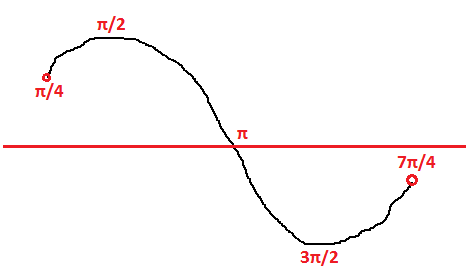# Function minima and maxima recap.

Came accross interesting problem in some GATE computer science paper.

Consider the function f(x) = sin (x) in the interval x ∈ [π/4, 7π/4]. The number and location(s) of
the local minima of this function are
(A) One, at π/2
(B) One, at 3π/2
(C) Two, at π/2 and 3π/2
(D) Two, at π/4 and 3π/2

Was quick to answer (B). Here is the plot of the graph, one of the minima is clearly 3π/2. However, in this case π/4 is a local minimum too (definitely clearer if we draw the function just within the ranges). So the answer is (D).Note that this is only true when the interval is closed i.e. including points π/4 and 7π/4. If the problem was x ∈ (π/4, 7π/4) then π/4 is NOT a local minima as its NOT part of the function domain. Also, no matter what point we pick close to π/4 as our local minimum there will be a number lower than that. In that case this function would have just one minimum at 3π/2.